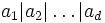# Structure theorem for finitely generated abelian groups

Jump to: navigation, search

## Statement

The structure theorem for finitely generated abelian groups states the following things:

1. Every finitely generated abelian group can be expressed as the direct product of finitely many cyclic groups (in other words, it is isomorphic to the external direct product of finitely many cyclic groups).
2. For any such expression, collect all the factors that are infinite cyclic and all the factors that are finite cyclic. Both parts are determined up to isomorphism by the isomorphism type of the original group. The former is termed the torsion-free part and the latter is termed the torsion part of the group.
3. For the torsion part: it can be written as a direct product of cyclic groups of prime power order. Further, for any two such expressions as a direct product, the number of groups of a particular prime power order is the same.
4. For the torsion part: there is a way of writing it as a direct product of cyclic groups of orders$a_1, a_2, \dots, a_d$, where$a_1 | a_2 | \dots | a_d$ with all$a_i$ positive integers. Moreover, the$a_i$s are completely determined by the isomorphism type of the group.

For a finite abelian group, the group has zero torsion-free part and the whole group as its torsion part. Parts (3) and (4) of the theorem still apply, and this version is termed the structure theorem for finite abelian groups.

In symbols, part (3) says that any finitely generated abelian group$G$ can be written as:$G \cong \mathbb{Z}^r \times \prod_{i=1}^l \mathbb{Z}/p_i^{k_i}\mathbb{Z}$

where$p_i$ are prime and$k_i$ are positive integers.$r$ is independent of the choice of expression. Further, the number of times any prime power$p^k$ occurs among the$p_i^{k_i}$ is independent of the choice of expressions.

In symbols, part (4) says that any finitely generated abelian group$G$ can be written as:$G \cong \mathbb{Z}^r \times \mathbb{Z}/a_1\mathbb{Z} \times \mathbb{Z}/a_2\mathbb{Z} \times \dots \times \mathbb{Z}/a_d\mathbb{Z}$

where the$a_i$ are positive, and$a_i$ divides$a_{i+1}$. Moreover,$r$ and$a_i$ are determined uniquely subject to these conditions.